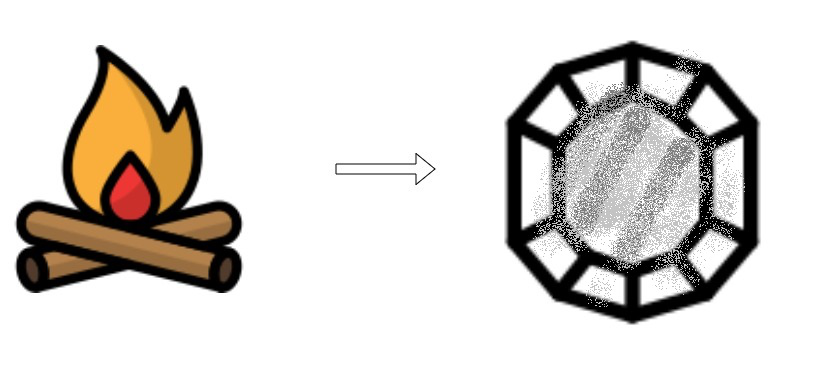# Conservation of Mass

• Last Updated : 14 Jul, 2021

A closed system conserves mass and energy contained and transferred within it. This principle is also known as mass balance and is maintained during any interval of time. The mass conservation principle is widely used in major domains such as chemistry, mechanics, and fluid dynamics.

Law of Conservation of Mass?

Attention reader! All those who say programming isn't for kids, just haven't met the right mentors yet. Join the  Demo Class for First Step to Coding Course, specifically designed for students of class 8 to 12.

The students will get to learn more about the world of programming in these free classes which will definitely help them in making a wise career choice in the future.

Mass is an isolated system. The law of conservation of mass states that the mass can neither be created nor destroyed in a chemical reaction. There is just a rearrangement in the atoms of substances for the formation of compounds. Therefore, the mass of the total system remains constant at any interval of time. This implies that the total masses of reactants is equal to the sum of masses of products and the masses of unreacted reactants. The law of conservation of mass is also termed as the principle of mass conservation.

Mass of the total enclosed system at the beginning of the reaction is equivalent to the mass at the termination of the reaction.

Formula for Law of conservation of massρ is the density

t is the time

v is the velocity

▽ is the divergence

Examples for the Law of Conservation of Mass

Production of Carbon Dioxide

For instance, the law of conservation of mass is widely observed in chemical reactions. For instance, if we consider the reaction of carbon with oxygen to produce carbon dioxide, it involves conservation of mass,
C (12g) + O2 (32g) = CO2 (44g)
Here we used 12 g of carbon and 32 g of oxygen as chemical compounds. After performing the reaction the amount of carbon –dioxide produced as the product will be 44g which is equivalent to the summation of the amount of carbon and oxygen used.

One molecule of water, with the chemical formula H2O has a molecular weight of 10. This is formed by the elements hydrogen having a molecular weight of 2 along with oxygen with a molecular weight equivalent to 8. Therefore, the mass is conserved.

Campfire

It may seem as though burning destroys matter, but the same amount, or mass, of matter, still exists after a campfire as before. Look at Figure 3.7.1 below. It shows that when wood burns, it combines with oxygen and changes not only to ashes but also to carbon dioxide and water vapor. The gases float off into the air, leaving behind just the ashes. Suppose you had measured the mass of the wood before it burned and the mass of the ashes after it burned. Also, suppose you had been able to measure the oxygen used by the fire and the gases produced by the fire. What would you find? The total mass of matter after the fire would be the same as the total mass of matter before the fire.It may seem as though burning destroys matter, but the same amount, or mass, of matter, still exists after a campfire as before.

Burning CandleThe burning of wood involves the usage of various elements like Oxygen, Carbon dioxide, water vapor, and ashes.

### Sample Questions

Example 1. If heating 10.0 grams of calcium carbonate (CaCO3) produces 4.4 g of carbon dioxide (CO2) and 5.6 g of calcium oxide (CaO), show that these observations are in agreement with the law of conservation of mass.

As we know that

Mass of the reactants = Mass of the products

10.0g of CaCO3 = 4.4g of CO2+5.6g of CaO

10.0g of reactant = 10.0g of products

Because the mass of the reactant is equal to the mass of the products, the observations are in agreement with the law of conservation of mass.

Example 2. Potassium hydroxide (KOH) readily reacts with carbon dioxide (CO2) to produce potassium carbonate (K2CO3) and water (H2O). How many grams of potassium carbonate are produced if 224.4 g of KOH reacts with 88.0 g of CO2? The reaction also produces 36.0 g of water.

The Law is also applicable to both chemical and physical changes. For example, if you have an ice cube that melts into a liquid and you heat that liquid up, it becomes a gas. It will appear to have disappeared, but is still there.

Example 3. Sodium carbonate reacts with ethanoic acid to form sodium ethanoate, carbon dioxide, and water. In an experiment, 5.3g of Sodium carbonate reacted with 6g of ethanoic acid to form 8.2g of sodium ethanoate, 2.2g of carbon dioxide, and 0.9g of water. Show that this data verifies the law of conservation of mass?

Solution:

We know,

Sodium carbonate + ethanoic acid = sodium ethanoate + carbon dioxide + water.

Substituting the masses of the compounds we have,

5.3g + 6g = 8.2g + 2.2g + 0.9g • 11.3 g= 11.3g

Hence, the conservation of mass is verified.

Example 4. Why should the chemical reactions be balanced?

Chemical reaction basically simulates a rearrangement of atoms. During the reaction, atoms (mass) can neither be created nor destroyed. In order to satisfy the chemical equations, equations must be balanced, since that states that matter cannot be produced or destroyed in a closed system.

Example 5. Who invented the Law of conservation of mass?

The principle of mass conservation was invented in 1789 by a French chemist, Antoine Lavoisier.

Example 6. Evaporation preserves the conservation of mass. Explain.